Courses

# Elementary Row & Column Operations - Matrices and Determinants, Business Mathematics & Statistics B Com Notes | EduRev

## B Com : Elementary Row & Column Operations - Matrices and Determinants, Business Mathematics & Statistics B Com Notes | EduRev

The document Elementary Row & Column Operations - Matrices and Determinants, Business Mathematics & Statistics B Com Notes | EduRev is a part of the B Com Course Business Mathematics and Statistics.
All you need of B Com at this link: B Com

## Elementary Matrix Operations

Elementary matrix operations play an important role in many matrix algebra applications, such as finding the inverse of a matrix and solving simultaneous linear equations.

## Elementary Operations

There are three kinds of elementary matrix operations.

1. Interchange two rows (or columns).
2. Multiply each element in a row (or column) by a non-zero number.
3. Multiply a row (or column) by a non-zero number and add the result to another row (or column).

When these operations are performed on rows, they are called elementary row operations; and when they are performed on columns, they are called elementary column operations.

## Elementary Operation Notation

In many references, you will encounter a compact notation to describe elementary operations. That notation is shown below.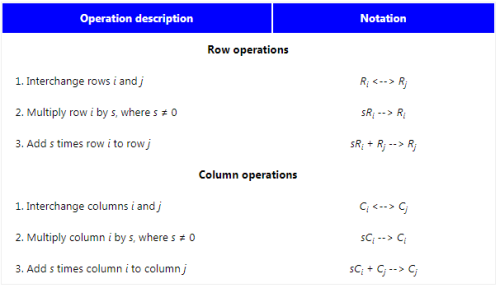## Elementary Operators

Each type of elementary operation may be performed by matrix multiplication, using square matrices calledelementary operators.

For example, suppose you want to interchange rows 1 and 2 of Matrix A. To accomplish this, you could premultiply A by E to produce B, as shown below.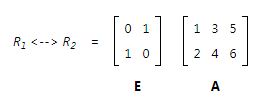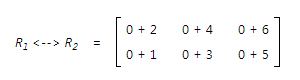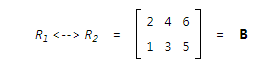Here, E is an elementary operator. It operates on A to produce the desired interchanged rows in B. What we would like to know, of course, is how to find E. Read on.## How to Perform Elementary Row Operations

To perform an elementary row operation on a A, an rc matrix, take the following steps.

1. To find E, the elementary row operator, apply the operation to an rr identity matrix.
2. To carry out the elementary row operation, premultiply A by E.

We illustrate this process below for each of the three types of elementary row operations.

• Interchange two rows. Suppose we want to interchange the second and third rows of A, a 3 x 2 matrix. To create the elementary row operator E, we interchange the second and third rows of the identity matrix I3.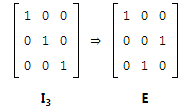Then, to interchange the second and third rows of A, we premultiply A by E, as shown below.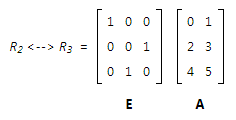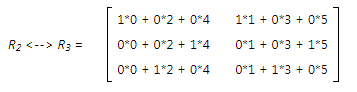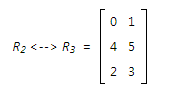Multiply a row by a number. Suppose we want to multiply each element in the second row of Matrix A by 7. Assume A is a 2 x 3 matrix. To create the elementary row operator E, we multiply each element in the second row of the identity matrix I2 by 7.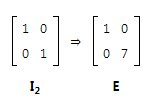Then, to multiply each element in the second row of A by 7, we premultiply A by E.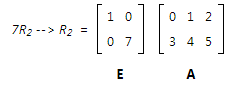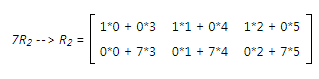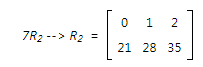• Multiply a row and add it to another row. Assume A is a 2 x 2 matrix. Suppose we want to multiply each element in the first row of A by 3; and we want to add that result to the second row of A. For this operation, creating the elementary row operator is a two-step process. First, we multiply each element in the first row of the identity matrix I2 by 3. Next, we add the result of that multiplication to the second row of I2 to produce E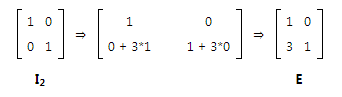Then, to multiply each element in the first row of A by 3 and add that result to the second row, we premultiply A by E.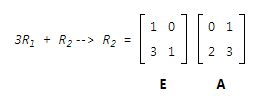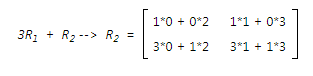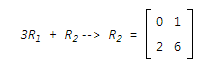## How to Perform Elementary Column Operations

To perform an elementary column operation on A, an r x c matrix, take the following steps.

1. To find E, the elementary column operator, apply the operation to an cc identity matrix.
2. To carry out the elementary column operation, postmultiply A by E
• Let's work through an elementary column operation to illustrate the process. For example, suppose we want to interchange the first and second columns of A, a 3 x 2 matrix. To create the elementary column operator E, we interchange the first and second columns of the identity matrix I2.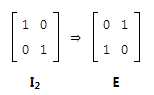Then, to interchange the first and second columns of A, we postmultiply A by E, as shown below.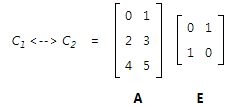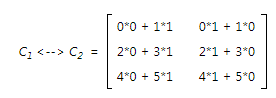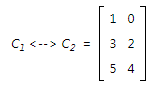Note that the process for performing an elementary column operation on an r x c matrix is very similar to the process for performing an elementary row operation. The main differences are:

• To operate on the rc matrix A, the row operator E is created from an rr identity matrix; whereas the column operator E is created from an cc identity matrix.

• To perform a row operation, A is premultiplied by E; whereas to perform a column operation, A is postmultiplied by E.

Problem 1

Assume that A is a 4 x 3 matrix. Suppose you want to multiply each element in the second column of matrix A by 9. Find the elementary column operator E.

Solution

To find the elementary column operator E, we multiply each element in the second column of the identity matrixI3 by 9.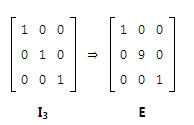Offer running on EduRev: Apply code STAYHOME200 to get INR 200 off on our premium plan EduRev Infinity!

122 videos|142 docs

,

,

,

,

,

,

,

,

,

,

,

,

,

,

,

,

,

,

,

,

,

,

,

,

;